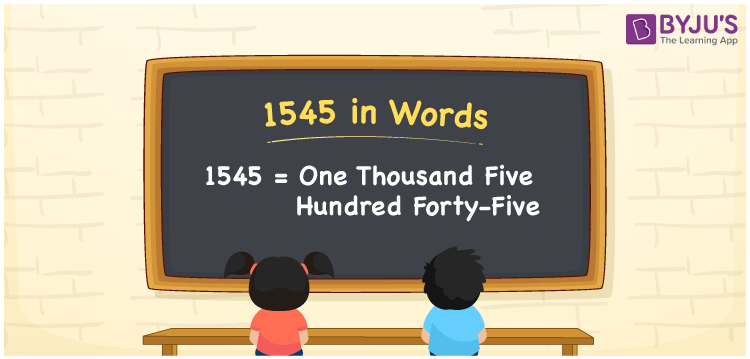# 1545 in Words

1545 in words can be written as One Thousand Five Hundred Forty-five. Students will be able to learn the conversion of 1545 in words which will help them understand the applications of numbers in our daily lives. If you buy a bracelet for Rs. 1545, then you can say that “I have bought a bracelet for One Thousand Five Hundred Forty-five Rupees”. The  number 1545 can be written in words using the English alphabet. The numbers in words can be grasped easily by the students using the resources given at BYJU’S. 1545 in English can be read as “One Thousand Five Hundred Forty-five”.

 1545 in words One Thousand Five Hundred Forty-five One Thousand Five Hundred Forty-five in Numbers 1545

## 1545 in English Words## How to Write 1545 in Words?

Students will learn about the conversion of 1545 into words from place value charts. The number 1545 has four digits. For 1545, the place value chart is prepared in a table form to help students understand it effectively.

 Thousands Hundreds Tens Ones 1 5 4 5

1545 in expanded form is explained in brief here:

1 × Thousand + 5 × Hundred + 4 × Ten + 5 × One

= 1 × 1000  + 5 × 100 + 4 × 10 + 5 × 1

= 1000 + 500 + 400 + 5

= 1545

= One Thousand Five Hundred Forty-five

Therefore, 1545 in words is written as One Thousand Five Hundred Forty-five.

1545 is a natural number that precedes 1546 and succeeds 1544.

1545 in words – One Thousand Five Hundred Forty-five

Is 1545 an odd number? – Yes

Is 1545 an even number? – No

Is 1545 a perfect square number? – No

Is 1545 a perfect cube number? – No

Is 1545 a prime number? – No

Is 1545 a composite number? – Yes

## Frequently Asked Questions on 1545 in Words

Q1

### How do you write 1545 in words?

1545 can be written as “One Thousand Five Hundred Forty-five” in words.
Q2

### Is 1545 an even number?

1545 is not an even number because it is not divisible by 2.
Q3

### How can One Thousand Five Hundred Forty-five be written in numbers?

One Thousand Five Hundred Forty-five can be written in numbers as 1545.